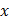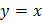# Equation (s) of the straight line(s), inclined atto the-axis such that the length of its (each of their) line segment (s) between the coordinate axes is 10 units is are a)b)c)d)## Question ID - 53594 :- Equation (s) of the straight line(s), inclined atto the-axis such that the length of its (each of their) line segment (s) between the coordinate axes is 10 units is are a)b)c)d)3537

(b,d)According to question. So,. Then equation of line isorNext Question :
 Ifandare two vertices of a triangle of area 4 sq. units, then its third vertex lies on a)b)c)d)